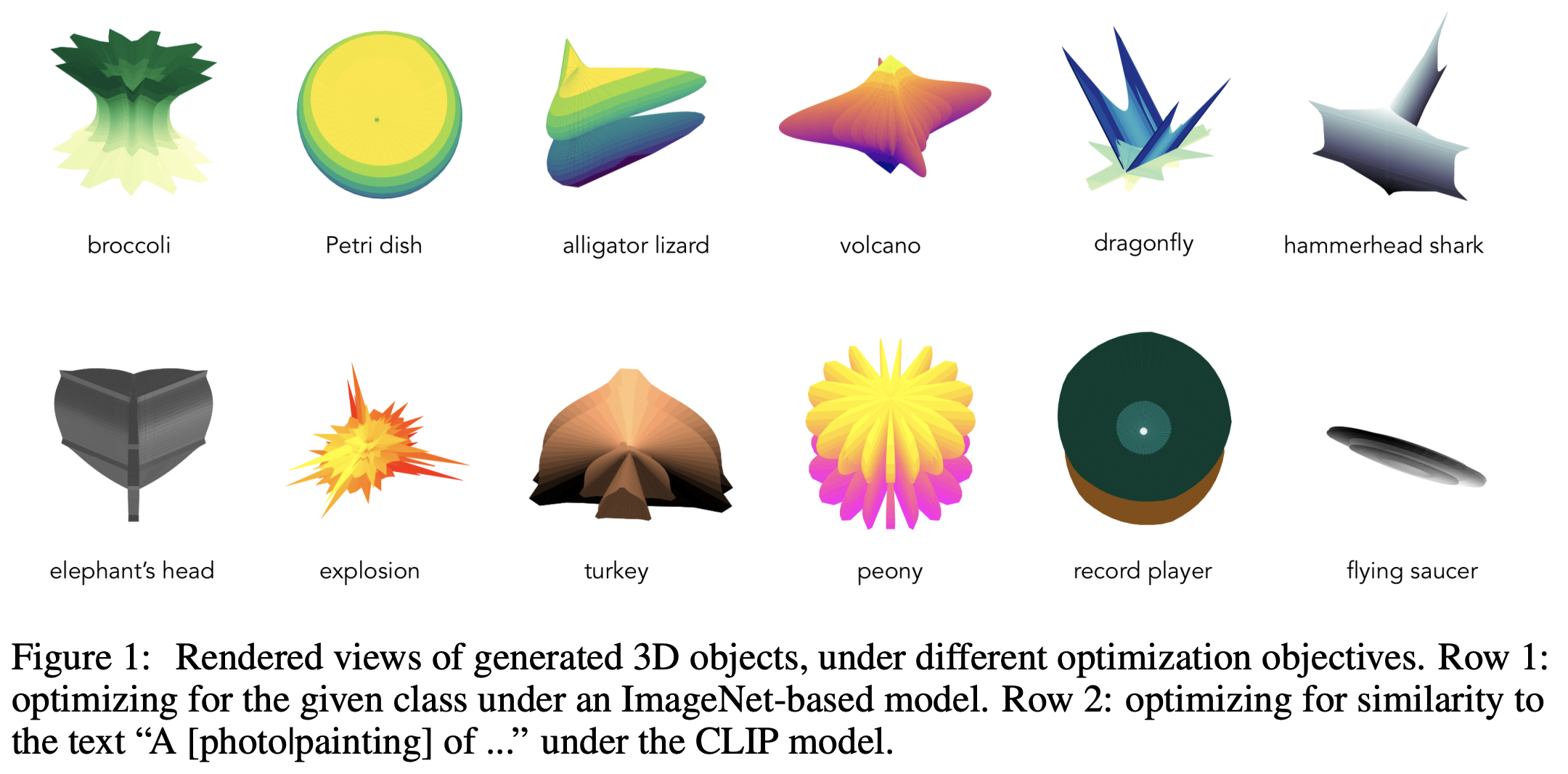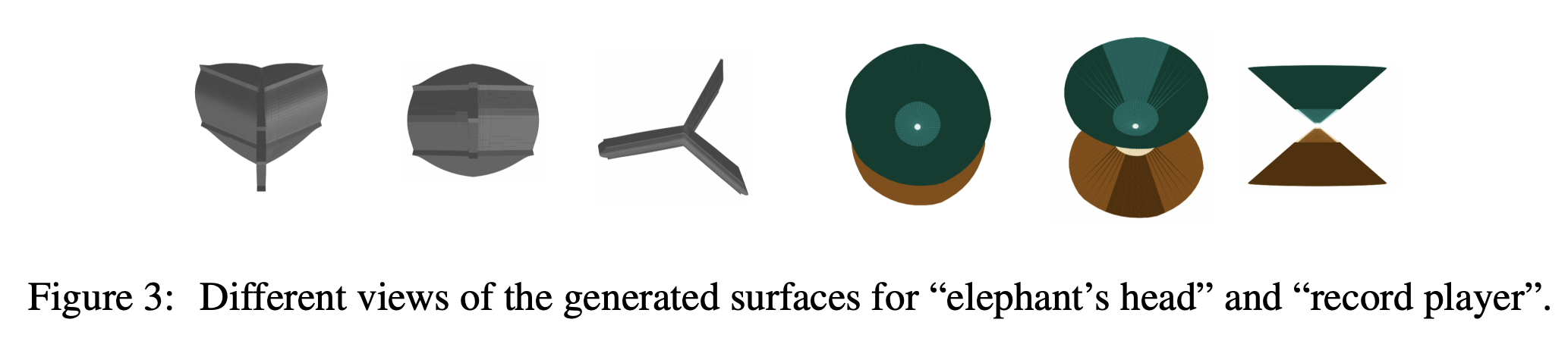# Evolving Evocative 2D Views of Generated 3D Objects

03 Nov 2021

Evolving Evocative 2D Views of Generated 3D Objects, Eric Chu, NeurIPS 2021 Creativity and Design Workshop

I came across a very interesting work that is purely for fun about generating 3D objects. People have been using image models such as BigGAN and CLIP to generate 2D artwork. But how about 3D artwork? Can an image model be applied to do so? The magic is to use a “superformula” of 3D model generation. The work introduces a “universal” equation to model a wide range of natural and abstract shapes. In short, all we need is 6 parameters $$m,a,b,n_1,n_2,n_3$$ to generate “any” 2D shapes:

$r(\varphi)=\left(\left|\frac{\cos \left(\frac{m \varphi}{4}\right)}{a}\right|^{n_{2}}+\left|\frac{\sin \left(\frac{m \varphi}{4}\right)}{b}\right|^{n_{3}}\right)^{-\frac{1}{n_{1}}}$

We can generalize it to 3D by using two instances of the superformula $$r_1$$ and $$r_2$$ by

\begin{aligned} &x=r_{1}(\theta) \cos \theta \cdot r_{2}(\phi) \cos \phi \\ &y=r_{1}(\theta) \sin \theta \cdot r_{2}(\phi) \cos \phi \\ &z=r_{2}(\phi) \sin \phi \end{aligned}

Now, we only need to figure out 12 parameters for the 3D shapes we want to generate. To map the generated shapes into image models, we also need viewpoint parameters: elevation, azimuth, and rotation. Thus, with 15 parameters, an image, as well as a 3D figure, are generated. To assess the image, the score can be either calculated by (1) the loss against a specific ImageNet class under a trained image classifier (e.g., MobileNetV3) or (2) the similarity to a caption under the image captioning model (e.g., CLIP). The whole process is not differentiable. Thus the optimization is done by a genetic algorithm-based optimization loop.

The results are quite interesting, as shown below. The superformula can create something reasonable:As the 3D objects are generated based on optimizing one viewpoint only, they are anamorphic, meaning the shapes can look completely different from other viewpoints:One can see this as a limitation that the 3D shapes do not generalize to different viewpoints, but also one can see it as an artistic style (it is called Richard Serra-style sculptures in the paper).